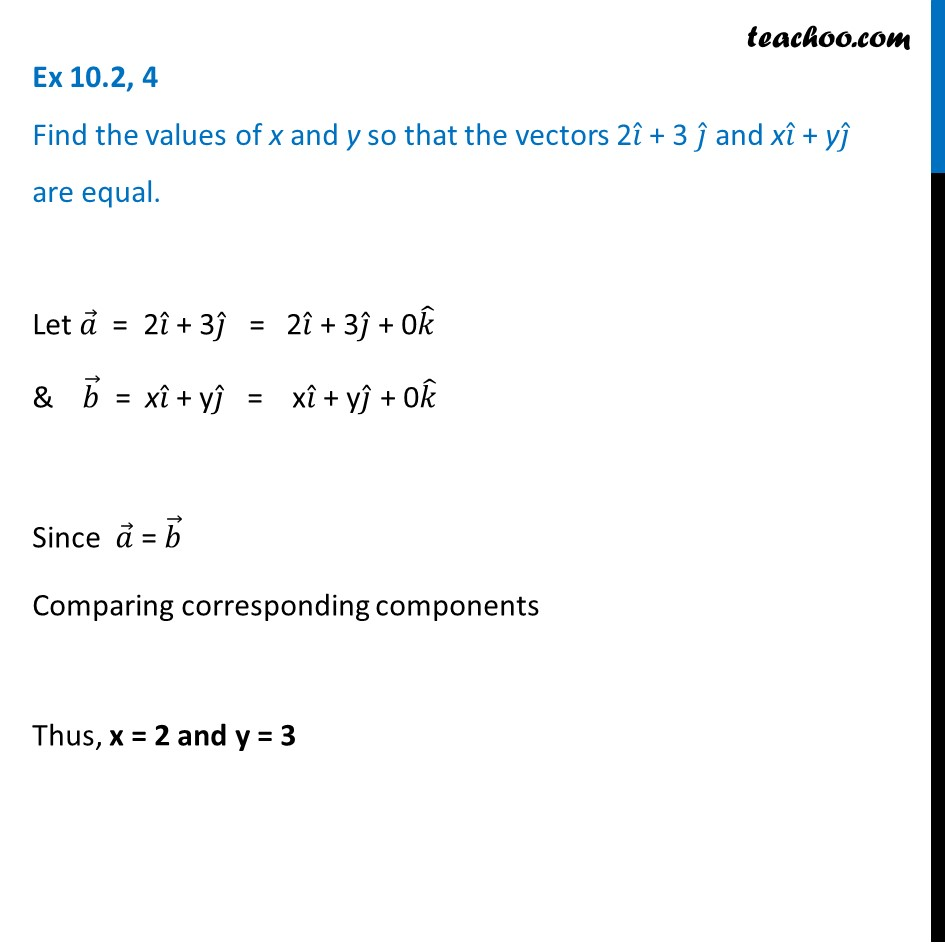Ex 10.2

Chapter 10 Class 12 Vector Algebra (Term 2)
Serial order wise### Transcript

Ex 10.2, 4 Find the values of x and y so that the vectors 2𝑖 ̂ + 3 𝑗 ̂ and x𝑖 ̂ + y𝑗 ̂ are equal.Let 𝑎 ⃗ = 2𝑖 ̂ + 3𝑗 ̂ = 2𝑖 ̂ + 3𝑗 ̂ + 0𝑘 ̂ & 𝑏 ⃗ = x𝑖 ̂ + y𝑗 ̂ = x𝑖 ̂ + y𝑗 ̂ + 0𝑘 ̂ Since 𝑎 ⃗ = 𝑏 ⃗ Comparing corresponding components Thus, x = 2 and y = 3